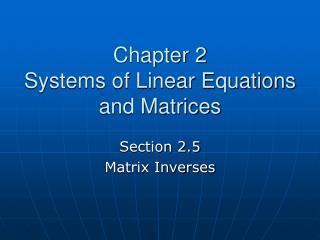# Chapter 2 Systems of Linear Equations and Matrices - PowerPoint PPT PresentationDownload PresentationChapter 2 Systems of Linear Equations and Matrices

Chapter 2 Systems of Linear Equations and Matrices
Download Presentation## Chapter 2 Systems of Linear Equations and Matrices

- - - - - - - - - - - - - - - - - - - - - - - - - - - E N D - - - - - - - - - - - - - - - - - - - - - - - - - - -
##### Presentation Transcript

1. Chapter 2Systems of Linear Equations and Matrices Section 2.5 Matrix Inverses

2. What is a Matrix Inverse? • The inverse of a matrix is comparable to the reciprocal of a real number. • The product of a matrix and its identity matrix is always the matrix itself. In other words, multiplying a matrix by its identity matrix is like multiplying a number by 1.

3. Multiplicative Identity • The real number 1 is the multiplicative identity for real numbers: for any real number a, we have a • 1 = 1 • a = a • In this section, we define a multiplicative identity matrix I that has properties similar to those of the number 1. We use the definition of this matrix I to find the multiplicative inverse of any square matrix that has an inverse.

4. Identity Matrix • If I is to be the identity matrix, both of the products AI and IA must equal A. • The identity matrix only exists for square matrices.

5. Examples of Identity Matrices

6. Determining if Matrices are Inverses of Each Other • Recall that a number multiplied by its multiplicative inverse yields a product of 1. • Similarly, the product of matrix A and its multiplicative inverse matrix A (read “A-inverse”) is I, the identity matrix. • So, to prove that two matrices are inverses of each other, show that their product, regardless of the order they’re multiplied, is always the identity matrix.

7. Example 1 • Prove or disprove that the matrices below are inverses of each other. a.) b.) c.)

8. Finding the Inverse of a Matrix

9. Row Operations on Matrices

10. Example 2 • Find the inverse, if it exists, for each matrix. a.) b.) c.)

11. Shortcut for Finding the Inverse of a 2 x 2 Matrix If a matrix is of the form then the inverse can be found by calculating: Note: ad – bc ≠ 0.

12. Example 3 • Find the inverse of the matrix below using the shortcut method.

13. Solution to Example 3 To find the inverse of the matrix use the formula and simplify.

14. Solution to Example 3 (continued)